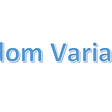# Visualizing Numerical and Categorical Features in one Graph

It’s an amazing way to see all data features in one graph. It gives us a clear picture about feature values. In this article, I’m going showing you an easy and a wonderful way to visualize data features whether categorical or numerical.

I will use Titanic dataset as an example to explore that.

First of all, we import essential libraries numpy, pandas, matplotlib and seaborn.

`import numpy as npimport pandas as pdimport matplotlib.pyplot as pltimport seaborn as sns`

`df = pd.read_csv(‘train.csv’)`

After that, take look for heading and show the shape of data.

`df.head()`
`df.shape`

Then, displaying information of data such as feature name, number of non-null values, data type.

`df.info()`

## Finally, we move to EDA aka exploratory data analysis.

All previous steps are considered preparing for EDA

Here and before we explore each feature versus other or target feature. I prefer and like seeing distribution and count of numerical and categorical features in one graph.

# Why this way is useful ?

In EDA, we do not need visualizing each feature.we just need the important features which they tell us about the data and give us meaning and some insights related to understand our business This question take us to other question.

# How can we explore all features in one graph?

Let us see how can we do that using Python. Step by step I be through with you until we plot graph for all features.

Matplotlib and Seaborn are basic libraries in python, they are used as widely range.

First Step
Create new function, name it plotting_features with one argument, name it data.

`def plotting_features(data):`

Second Step
Create docstring for your function to help programmers better understand the intent and functionality of your codes inside function.

`‘’’Return to distribution of numerical features and count of categorical features, specific features will ignore thembecause they do not give us any meaning.‘’’`

Some features specifically categorical features with large unique values they do not give us any meaning if we plot them such as PassengerId, people name, Ticket and Cabin, hence we ignore them from our graph.

Third Step
Create new two list. Fist list, name it ignored_cols then, insert all features which will be ignore into this list. Second list, name it cols then, insert all features which would be plotted into this list

`ignored_cols = [‘PassengerId’,’Name’, ‘Ticket’, ‘Cabin’]cols = [ col for col in data.columns if col not in ignored_cols]`

Forth Step:
Create a variable, name it number of rows then, put number of rows in it.

`nrows= int(np.ceil(len(cols)/2))`

For example:
10/3 = 3.333
np.ceil(10/3) give us 4.

I prefer two plots in each row of subplot graph that give us an appropriate size to see all details of each plot.

But you can change it “subplots” to 3, 4 or so on in one row.

`nrows= int(np.ceil(len(cols)/2))`

Fifth Step
Create two variable, name them fig and ax, separate them with comma then put subplots function in it and set first argument with nrows, second argument with ncols, third argument with figsize and forth argument with constrained_layout as True to fit a plot size of axes.

`fig, ax = plt.subplots(nrows=nrows, ncols=2, figsize=(12,8), constrained_layout=True)`

Sixth Step
Create variable, name it ax then create a function by using ax.ravel() with default arguments to convert axis of plots from multi array ‘matrix to one array to be an easy to set each axis

`ax = ax.ravel()`

For example:

Seventh Step
Create for loop with index in range of features length, then create if-else statement. Hence if data type of feature is an object string or length of unique value less than ten, create count plot function, put in it two keyword arguments, first one y = feature with specific index and second one ax = axis with same index. After that, set axis title with name of feature, else, create hist plot function, put in it two keyword argument first one x = feature with specific index and second one ax = axis with same index.

`for i in range(len(cols)):    if (data[cols[i]].dtypes == ‘object’) |       (len(data[cols[i]].unique().tolist()) < 10):                    sns.countplot(y = data[cols[i]], ax=ax[i])       ax[i].set_title(f’{cols[i]} count’)    else:       sns.histplot(x = data[cols[i]], ax=ax[i])       ax[i].set_title(f’{cols[i]} distribution’);`

Eighth Step
Now, our function become ready, call function and set an argument name the name of data then run it.

`plotting_features(df)`

You can see the whole function below, write and run it to see the plot of features.

`def plotting_features(data):        '''    Return to distribution of numerical features and count of     categorical features, specific features will ignore them,    because they do not give us any meaning.        '''     ignored_cols = [‘PassengerId’,’Name’, ‘Ticket’, ‘Cabin’]    cols = [ col for col in data.columns if col not in ignored_cols]     nrows= int(np.ceil(len(cols)/2))    fig, ax = plt.subplots(                        nrows=nrows,                         ncols=2,                         figsize=(12,8),                           constrained_layout=True)    ax = ax.ravel()     for i in range(len(cols)):        if (data[cols[i]].dtypes == ‘object’) |           (len(data[cols[i]].unique().tolist()) < 10):                         sns.countplot(y = data[cols[i]], ax=ax[i])            ax[i].set_title(f’{cols[i]} count’)         else:            sns.histplot(x = data[cols[i]], ax=ax[i])            ax[i].set_title(f’{cols[i]} distribution’);plotting_features(df)`

Finally, you have given a good idea how can you plot all features in one graph in details? try it and enjoy when you write code.

Wait wait wait

It is a good and nice idea but …..

What about if we have large of features?

For example, in house prices dataset we have more than eighty features.

## How can we deal with that?

Ok, that is a good question.

We can divide them into groups then, plot each group in one graph.

let us look at the below figure, we taken the first ten featuers, plotted them in one graph, then next ten features and so on.

--

--

--

## More from Abdulaziz Alsulami

A self Learner interested for AI & Python, love reading, write his thoughts to his own way and like presenting knowledge to world in picture which he like.

Love podcasts or audiobooks? Learn on the go with our new app.

## Cohort 9 Student Spotlight: Meet Daniel Blessing## Data Science: Need and Scope## How to Jitter Well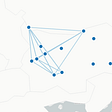## Performing Analysis of Meteorological Data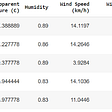## Data Careers in Tech## UDAF and Aggregators: Custom Aggregation Approaches for Datasets in Apache Spark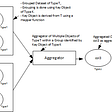## Abdulaziz Alsulami

A self Learner interested for AI & Python, love reading, write his thoughts to his own way and like presenting knowledge to world in picture which he like.

## The best beginner’s guide on K-Means Clustering.## Performing Analysis of Meteorological Data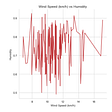## Random Variables